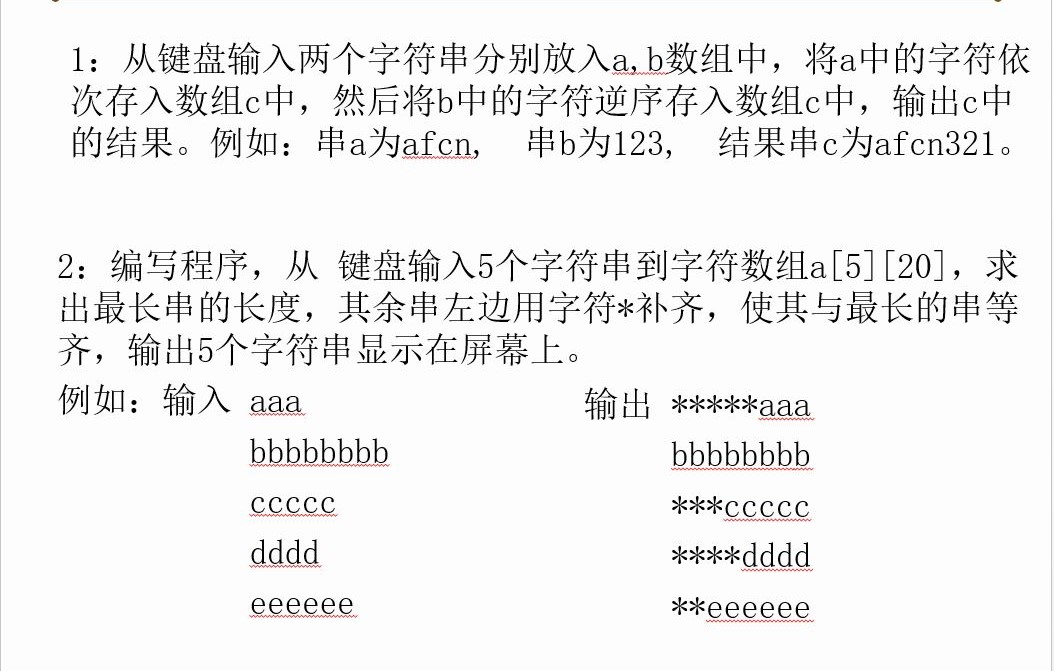2020-03-30 17:29

# 两个C语言编程该怎么写？• 点赞
• 写回答
• 关注问题
• 收藏
• 邀请回答

#### 1条回答默认 最新

•qtchen_1988 2020-03-30 19:04
已采纳

第一题：

``````#include <stdio.h>
#include <string.h>

#define BUF_SIZE 20

int main()
{
char a[BUF_SIZE];
char b[BUF_SIZE];
char c[BUF_SIZE*2];
memset(a,0x0,BUF_SIZE);
memset(b,0x0,BUF_SIZE);
memset(c,0x0,BUF_SIZE*2);
char t;
int i = 0;
while ((t=getchar()) != '\n')
a[i++] = t;
i = 0;
while ((t=getchar()) != '\n')
b[i++] = t;
int k;
int a_l = strlen(a);
for(k=0;k<a_l;k++)
c[k] = a[k];
int b_l = strlen(b);
int n = b_l;
for(i=0;i<b_l;i++)
c[k+i] = b[--n];
printf("c=%s\n",c);

return 0;
}

``````

第二题：

``````#include <stdio.h>
#include <string.h>

int main()
{
char a = {};
int max_leng = 0;
int i = 0;
while (i<5) {
char t;
int j = 0;
while ((t=getchar()) != '\n')
a[i][j++] = t;
if(j > max_leng)
max_leng = j;
i++;
}
for(i=0;i<5;i++)
{
int l = strlen(a[i]);
int k = 0;
int n = 0;
while (k<max_leng) {
if(k < (max_leng-l))
printf("*");
else
printf("%c",a[i][n++]);
k++;
}
printf("\n");
}
return 0;
}

``````
点赞 打赏 评论